# Voltorb Flip Guide

In the non-Japanese versions of HeartGold and SoulSilver, there is a new minigame replacing the old slot machines, Voltorb Flip. Unlike the slots, this is actually a logic puzzle that requires some thinking, and thus I thought it would be appropriate to write a guide about it.

## How It Works

Well. You have a game board. It looks somewhat like this.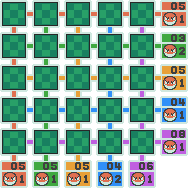There are twenty-five green squares, and these squares each contain either a Voltorb (equivalent to zero) or the numbers 1, 2 or 3. When you tap a square, you will flip it over to see what it contains.

At any point in the game, your score is equal to all the numbers you've flipped over multiplied together. This inevitably means that once you flip over a zero, you've lost all your points and can never get them back. But just to make the imagery a bit stronger, the zeroes are Voltorb that will violently explode and end your game immediately (after all, there's no point in continuing when your score has a multiplier of zero in it). In other words, you don't want to flip over the Voltorb. Meanwhile, the 1s don't really matter; multiplying your score by one just keeps it the same. Therefore, the goal of the game is to flip over all the 2s and 3s on the table, and once you've done that, no matter how many 1s are left, you'll get your payout and advance to the next level. If you flip over a Voltorb at any point, however, you'll lose all your winnings for this round, and if the number of squares you flipped before this happened is less than the level you're currently on, you'll drop back to the level corresponding to the number of flipped squares (though of course, since there is no level zero, if the first thing you flip over is a Voltorb you'll be sent to level one instead).

So how do you know which squares to flip? That's where those numbers on the right and bottom sides come in. The top number in each of the squares is the sum of all the numbers in that row or column, while the bottom number is the number of Voltorb in that row or column. And from this, you need to work it out. You've got a memo pad to assist you, which allows you to give any square markers corresponding to Voltorb, 1, 2 or 3.

## So How Do I Win?

Logic, my friend. There are several rules you can follow to narrow it down for you. Since it would be tiring to type "row/column" every time, from now on I will use "row" to refer to both horizontal rows and vertical columns. They are functionally interchangeable, so all the same rules apply vertically. Likewise, though all the examples display horizontal rows, they could just as well be vertical.

### The Basic Rules

#### Rule #1: No Voltorb

Simple and straightforward here: basically, the first thing you should do is always to look at all the Voltorb numbers and check if there are any rows with no Voltorb at all.

If a row has no Voltorb, it is obvious it is safe to just flip over the whole row. Therefore, you should do just that if you have any zero-Voltorb rows. You may notice that a 5/0 column will only give you ones anyway and think it's not worth the bother to flip it over, but do it; it will both prevent you from falling too far if you lose and serve as a reminder that you know what these squares contain.

Example: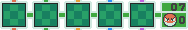becomes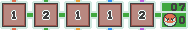#### Rule #2: Only Voltorb

Almost as simple and straightforward: if a row is 0/5 - that is, it has five Voltorb and no actual numbers - you naturally don't want to flip any of that. But no, that does not mean you just leave it alone and think about something else. Bring up the memo pad (X or the button at the top right) and mark all the squares in that row with a Voltorb. This will tell you that you know these squares are Voltorb.

Example: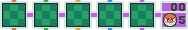becomes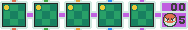#### Rule #3: Total Five

We're getting to slightly less obvious now, but nonetheless still straightforward. If any row's total and Voltorb numbers add up to five, then it's clear that that row contains only Voltorb and ones. This is because five (the total number of squares in the row) minus the Voltorb number gives the number of squares containing some number, and if that number is the same as the total, they all inevitably have to be 1s. You could be misled to think this means the row is irrelevant and you can ignore it, but don't; yet again, open the memo pad and mark all the (remaining, if you've already flipped some over or marked them as definitely Voltorb) squares in the row with both the Voltorb and 1 marks, to say that they're either Voltorb or 1s but not 2s or 3s.

Example: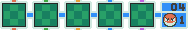becomes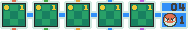#### Rule #4: Four Voltorb

If any row has four Voltorb, you know that only one of its squares is a number. This inevitably means that the total number is equal to that one number to be found in the row, and therefore, for every square in the row, you can eliminate all possibilities other than Voltorb and the total number. The 1/4 case is of course just a special case of the above, but for 2/4 and 3/4, this gives quite useful information that is often overlooked.

Example: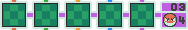becomes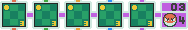#### Rule #5: Total Six

People really don't seem to point this out a lot, but you can rule out 3 for any square in a row whose total and Voltorb numbers add up to six. The reason for this is that if you take away the Voltorb squares, this leaves you with one less square than the total number, and the only way this can happen is if one of those squares is a 2 and the others are 1s. And yes, this means it is useful to mark those squares.

Example: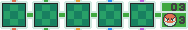becomes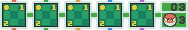#### Rule #6: Too High for 1s

In a similar fashion to the Total Six rule, sometimes you can eliminate the possibility of 1s in the row. This happens if the number of number squares (i.e. five minus the Voltorb number) is less than or equal to a third of the total number plus one, i.e.:

• there is one number square (four Voltorb) and the total number is at least two (technically a special case of the Four Voltorb rule)
• there are two number squares (three Voltorb) and the total number is at least five
• there are three number squares (two Voltorb) and the total number is at least eight
• there are four number squares (one Voltorb) and the total number is at least eleven
• there are five number squares (no Voltorb) and the total number is at least fourteen (I must confess I don't know if this can actually possibly happen in the game, but hey)

Note that even if this doesn't apply, it is still possible there are no 1s in the row; it's just that you can't rule out that there might be a one. This is basically when the row is either all 3s or one 2 with the rest 3s; if there are at least two 2s, you can't immediately outrule that those two 2s could be a 1 and a 3 instead. Note also that this means you can eliminate the possibility of 2s as well if the total number is just one higher than the minimums indicated above, since that means the row is all 3s and Voltorb.

Example: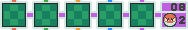becomes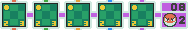#### Rule #7: Eliminate the Impossible

Remember that when you place markers, you are eliminating the numbers you don't mark. That also means you're not going to add any of them back in unless you realize you made a mistake in eliminating them in the first place. Basically, if you've already got some markers on a square and you find out something about that square's row or column, this should only result in the removal of markers, not new ones being added. You can compound what you know from the row and column this way, giving you still more information about a square.

Example: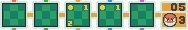becomes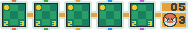#### Rule #8: Whatever Remains...

Whenever you eliminate possibilities, you may be uncovering the mysteries of an entire row. If a row must have at least X 1s, 2s or 3s, then any time you have exactly X squares left with that number as a possibility, you can safely flip those squares over, because that number simply must be there. Be sure you're not making a mistake before you do that, though - double-check whether the row truly must have that many of that number still unflipped and whether the other squares truly have it eliminated. It's always nasty to trip a Voltorb by accident on a square you thought was safe.

So when must a row have at least X 1s, 2s or 3s? Use the following rules, given that we call the number of number squares (not-Voltorb) N and the total number for the row T:

• In a row where T < 2N, there must be at least 2N-T 1s. (So if there are three number squares and the total for the row is four, there must be at least 2*3-4 = two 1s.)
• In a row where T > 2N, there must be at least T-2N 3s. (So if there are four number squares and the total for the row is nine, there must be at least 9-2*4 = one 3.)
• In a row where T's evenness is opposite that of N (that is, if N is even T is odd, or if N is odd T is even), there must be at least one 2. (So if there are three number squares and the total for the row is six, there must be at least one 2.)

Note that there can be more 1s, 2s and 3s than indicated here; these are just minimums that must be present in the row when these rules apply.

Example: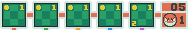becomes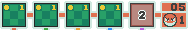Another example: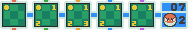becomes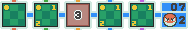(Note that a square containing all four markers is equivalent to an unmarked square here; I personally like to fill out the whole board with 0123 markers on every remaining square after I've applied the five most basic rules, but you don't have to do that if you're satisfied with knowing an empty square means you've eliminated nothing.)

#### Rule #9: Cannot Be Voltorb

Sometimes, even though you can't tell exactly which number a square is hiding, you can tell that it must be some number - that is, it can't be a Voltorb, and thus it is safe to flip it over.

This happens when, out of all the possible combinations of numbers the row could have to reach the given total, they all need this square to be included, on the basis of what has been eliminated in the other squares. This explains itself best with an example. Say you have a row like this: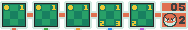It's total seven so that doesn't help us; there are Voltorb but not four or five of them; 1s, 2s and 3s are all possible; and while there must be at least one 1, that doesn't help us here since we haven't eliminated 1s from any of the squares, while we have no guarantees about the presence of either 2s or 3s. Using just the above rules, we'd be at a loss here. But don't give up just yet.

We have three number squares and must get a total of five from them. How many ways can this happen? There are two possibilities - 1-2-2, or 1-1-3.

Notice anything? If this row has a 1 and two 2s, there are only two possible places those 2s can go - they must be the fourth and fifth squares. Meanwhile, if this row has two 1s and a 3, there is only one place that 3 can go - the fourth square. So either way, the fourth square must be either a 2 or a 3! And thus, it cannot possibly be a Voltorb. Therefore, we can safely flip it over. Even better, whether this number happens to be a 2 or a 3, we find out more about the row - if it's a 3, the other numbers in the row are both 1s and so we can eliminate the 2 from the fifth square, while if it's a 2, we know there's another 2 and that it must be the fifth square, allowing us to flip it over as well.

If you don't want to have to work out the possible combinations every time, here's a quick cheat sheet of the stuff you need this rule to solve - in the rest, there is only one possible combination and rule eight can tell you everything you need:

Four Number Squares
Total six: 1-1-2-2 or 1-1-1-3
Total seven: 1-1-2-3 or 1-2-2-2
Total eight: 1-1-3-3, 1-2-2-3 or 2-2-2-2
Total nine: 1-2-3-3 or 2-2-2-3
Total ten: 1-3-3-3 or 2-2-3-3
Three Number Squares
Total five: 1-2-2 or 1-1-3
Total six: 1-2-3 or 2-2-2
Total seven: 1-3-3 or 2-2-3
Two Number Squares
Total four: 1-3, 2-2

It can be a little time-consuming to work this out, so I recommend only doing it if none of the other rules are giving you anything - hence my placing this as the last rule.

### Generalizing

Here's the thing. The nine rules above don't just apply to the rows as we have them at the beginning. You can apply the same reasoning after you've flipped over a square by simply subtracting the number you've flipped from the total and then treating the row as if it were only four squares, with the appropriate tweaks to the rules. For instance, say you have a row like this: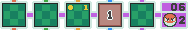None of our current rules tell us how to deal with this. However, because we've flipped over that 1 already, we can mentally pretend this row looks like this: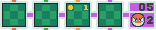And hey, what's that? We have two number squares and a total of at least five! (Remember, the Voltorb counts listed in the Too High for Ones rule assume a total of five squares in the row - with four in our imaginary row, two Voltorb leave two number squares.) So rule six tells us the remaining squares in this row can only be Voltorb, 2s or 3s...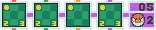And voilà, we've not only eliminated 1 from those other three squares, but also confirmed without a doubt that that third one is a Voltorb. Score!

Let's take another example. Say we uncover a 2 in this row: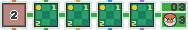Mentally remove it and subtract from the total like we did above, and...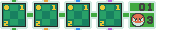Gasp! Is that the Total Five rule I see? No, it's not total five, but it's total four, and now there are only four squares in this imaginary row, so the same principle applies. We can eliminate all those 2s, giving the whole row Voltorb-one markers. Note that this is also the Four Voltorb rule generalized to apply to reduced rows - for four squares in a row, it's essentially the "Three Voltorb" rule. Either one of them will give you the same result, since this is an overlapping case.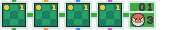Likewise, the Total Six rule applies to a total of five when you have four squares and, just like with five squares, gives you the ability to eliminate 3s from all the remaining squares where it applies.

You can of course do the same thing when you've flipped over more than one number in the same row - again, just subtract them all from the total and pretend the row is only however many squares remain when you've done that. And you can do the same when you've confirmed for sure that a square is a Voltorb by mentally subtracting from the Voltorb count instead of the total number. This will simplify your rows and is often the key to solving the puzzle.

In general, every time you've flipped over a square or eliminated some possibilities, reevaluate both that square's row and column in light of the new changes, reducing them to fewer squares as necessary and applying all nine rules in order. It may seem like this will take absolute ages, but you start to see very quickly whether most of the rules apply - the first five need only a glance at the numbers (or mental numbers), while the latter four are something you get used to applying after a while.

### Educated Guessing

Don't you love how we've come this far in the guide with absolutely no mention of guesswork? And people complain this game is just luck like the slots. Pssh.

But, well, in most games (not all - I've gotten through many rounds on logic alone without guessing at all), eventually none of the nine rules will lead you anywhere with any row or column. You'll just have some identically marked squares and absolutely no way to tell which of them hides the Voltorb. So what do you do then?

The most basic rule for guessing is to flip over a square where two rows with few Voltorb and a high total intersect - it's fairly likely to be a 2 or 3 and unlikely to be a Voltorb. However, do this with reduced rows in mind, not the totals displayed on the sides - if there's a 9/1 column, you still won't want to guess a square in it if you've already flipped over two 3s and a 2. You should also pretty much never guess a square marked Voltorb-1 - too much risk and too little potential reward (some will balk and ask what potential reward, but it will be insurance against falling too many levels, and revealing a 1 can in various circumstances give you valuable information about a row - it's just rare that you couldn't get the same information at the same risk by revealing a potentially valuable square).

Remember that just because you get a Voltorb sometime when you guess, it doesn't mean the guessing rules are useless or it's just luck or whatever your disgruntled mind might throw up when you're exasperated. Anything probability-based will have you get unlucky sometimes, but you really can make a pretty good guess, and for every time you lose when making a good guess, you'll also win several times.

### Quitting

The game gives you a Quit option you can use at any time, which will count as a loss (and bump you down some levels if you haven't flipped over enough squares to stay) but let you keep the coins you've earned in that round so far.

This isn't really as useful as you might think - your chances rarely get worse than fifty-fifty when guessing, while the reward if you get it right will inevitably at least quadruple your winnings, making continuing easily worth it from a game theory standpoint. But sometimes you just want to earn some coins and you're on a pretty good streak, and then you can decide to quit when you get to a difficult guess to avoid losing it all. Just keep in mind it usually pays to guess, no matter how much your intuition is convinced otherwise.

## Sample Games

To make this all a bit clearer, I'll go through a whole game for you, the way I'd solve it. This is an actual run from my SoulSilver; as it happens it's level four, but all the same principles apply at both the lower and higher levels.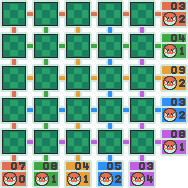To start with, let's apply Rule #1, No Voltorb. As you can see, the first column has no Voltorb in it, so we can safely flip it over.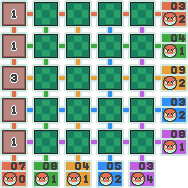There are no five-Voltorb rows or columns, but there are several with a total of five, so let's mark those.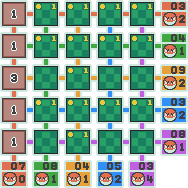Now, the fifth column has four Voltorb and its total is three, so this means we can eliminate 1s and 2s from every square in it, and since it intersects so nicely with three Voltorb-1 rows, that leaves us with...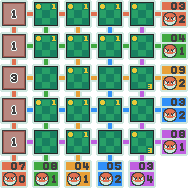Notice how the second row has only one Voltorb, and here we've confirmed that the fifth square in it is definitely a Voltorb - that means the rest of the row can be safely flipped over, even though it's only 1s. To phrase this in the rules given above, we can reduce it to a 3/0 row (after subtracting both the confirmed Voltorb and the confirmed 1), which falls under the No Voltorb rule.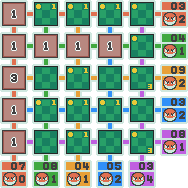Because I prefer to work like that, I'm going to fill in the empty squares with 0123 markers to make things plainer: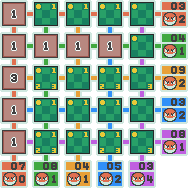So now I happen to notice that the second-column 0123 squares Cannot Be Voltorb, in accordance with Rule #9 - when we reduce it, we find that both possible combinations that make a seven in three number squares require at least two 2s or 3s. In fact, it is plain that both of them must be 3s. So we can safely flip them over as well.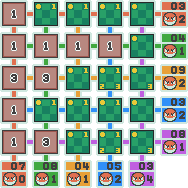Take a look at the third row - if we reduce it, it becomes a three-square 3/2 row, which falls under #4, the Four Voltorb rule (here "Two Voltorb", for a row of three squares); the only non-Voltorb square must be a 3. So we can eliminate the third square altogether, and eliminate the 1 and 2 from the fourth square.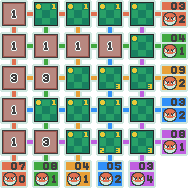We can reduce the fifth row to a three-square 4/1 row. And now we see that there are only two combinations that will yield a four in two number squares, 2-2 and 1-3 - and 2-2 is not possible, since there is only one square that can be a 2. Thus, we can eliminate that 2 altogether.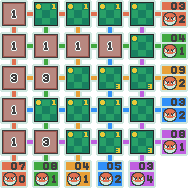Now we're in trouble. If we look at the fourth column, we can reduce it to a four-square 4/2 row, but that doesn't tell us anything new. We still have two possibilities for 3s in this column and two in the fifth column, and there is no way to tell which is which. It's guessing time!

So how do we guess? We know that in the third row, there simply must be a Voltorb in either the fourth or fifth square - it's a fifty-fifty. Likewise, if we look at the fifth column, it's a fifty-fifty whether the last Voltorb lies in the third or fifth square. However, the fifth row makes it possible for the one Voltorb to be in any of the last three spaces, and the fourth column has its two Voltorb in any of four spaces with one possibility (the third and fifth squares being Voltorb) eliminated thanks to the column needing a 3. This makes the fifth-row, fourth-column square our best guess. So let's tap it and hope for the best...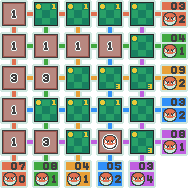...and it's a Voltorb. Let's face it: sometimes you just guess wrong and lose. But see how far we got on logic alone? And if we'd guessed correctly, a couple of simple steps of logic would have revealed the final remaining 3 as well, resulting in nine times the reward that we lost by guessing wrong rather than quitting. Plus, the good thing about losing on endgame guessing is that you'll uncover enough squares first so that you don't get bumped down a level, which is always a plus - you can simply try again.

Let's take another sample game. This one's level five. Here's my board: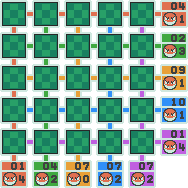As always, we can start with flipping over all the squares in that no-Voltorb column and marking the total-five, total-six and four-Voltorb rows before marking the rest with all four markers (again, you don't have to, but I do it):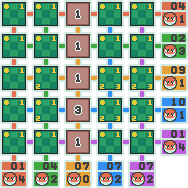Now look at the third row, the 9/1 one. Since we've flipped over a 1, we can reduce it to a four-square 8/1 row, and... that makes it Too High for 1s, since there are three number squares and a total of eight. So let's eliminate the 1s there: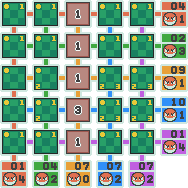...but wait. Is that a confirmed Voltorb? In a one-Voltorb row? Score! We're safe to flip the entire rest of that row over now: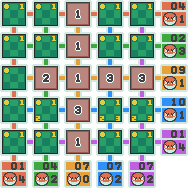Sweet; the score is up to 54 and we've revealed quite a bit of the board. Even better, look at what this reveals to us about the second column: we can reduce it to a four-square 2/2, and that's the four-square equivalent of a Total Five, so let's eliminate the 2 from that fourth square...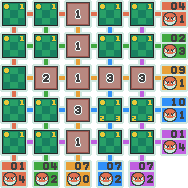And this leaves us with only two potentially valuable squares left - and even better, they're demonstrably both valuable. Why? One way to look at it is to reduce the fourth row to a four-square 7/1 row and realize that both of them Cannot Be Voltorb - both possible three-number-square combinations for a total of seven require at least two 2s or 3s. Another way to look at it would be to simply glance at the fourth and fifth columns - a 3 in both of those squares is the only way they can total seven. So we happily flip them over and handily win 486 coins on logic alone, with not a single guess anywhere in the process.

I won't lie - it's not very common that you don't have to guess at all, and many games have you guessing several times, with each successful flip revealing little, until you trip a Voltorb. But it really is possible to play a game that doesn't require any guessing, and not uncommon for there to be only one or two educated guesses during the course of the game.